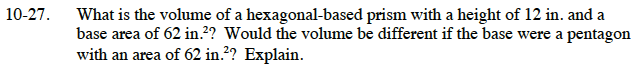### Home > CC3 > Chapter Ch10 > Lesson 10.1.2 > Problem10-27

10-27.

What is the volume of a hexagonal-based prism with a height of 12 in. and a base area of 62 in.2? Would the volume be different if the base were a pentagon with an area of 62 in.2? Explain. Homework Help ✎Volume of a prism = (area of base)(height)

Even if the base shape changes, if the area of the base and height remains the same, would the volume be different?

The volume of each prism would be 744 in³. The shape of the base does not matter as long as the base area is the same.